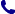• Kontakt
• Newsletter Zapisz się po informacje o nowościach i specjalnych ofertach. Administrator danych: ABE-IPS sp. z o.o.
Wybierz interesujące Cię kategorie
Wyrażam zgodę na przetwarzanie moich danych osobowych w celach marketingowych, tym samym akceptuję Regulamin Newslettera i Politykę prywatności.
Wyrażam zgodę na przesyłanie informacji handlowych drogą elektroniczną.
Wypisz się
• Klienci instytucjonalni
• Dostawa
• O nas
Dostęp on-line

# Książki

0.00 PLN
Schowek (0)
 Schowek jest pusty

## Oxford IB Diploma Programme: IB Mathematics: applications and interpretation, Standard Level, Print and Enhanced Online Course Book Pack

 Wydawnictwo Oxford University Press Data wydania 2019 Liczba stron 672 Forma publikacji zestaw Poziom zaawansowania Dla szkół wyższych i kształcenia podyplomowego Język angielski ISBN 9780198426981 Kategorie Matematyka
548.10 PLN (z VAT)
\$123.29 / €117.51 / £102.01 /
Produkt na zamówienie
Dostawa 3-4 tygodnie
Ilość
Do schowka

## Opis książki

Featuring a wealth of digital content, this concept-based Print and Enhanced Online Course Book Pack has been developed in cooperation with the IB to provide the most comprehensive support for the new DP Mathematics: applications and interpretation SL syllabus, for first teaching in September 2019. Each Enhanced Online Course Book Pack is made up of one full-colour, print textbook and one online textbook - packed full of investigations, exercises, worksheets, worked
solutions and answers, plus assessment preparation support.

Oxford IB Diploma Programme: IB Mathematics: applications and interpretation, Standard Level, Print and Enhanced Online Course Book Pack

## Spis treści

Measuring space: accuracy and 2D geometry

1.1: Measurements and estimates

1.2: Recording measurements, significant digits and rounding

1.3: Measurements: exact or approximate?

1.4: Speaking scientifically

1.5: Trigonometry of right-angled triangles and indirect measurements

1.6: Angles of elevation and depression

Representing space: non-right angled trigonometry and volumes

2.1: Trigonometry of non-right triangles

2.2: Area of triangle formula. Applications of right and non-right angled trigonometry

2.3: Geometry: solids, surface area and volume

Representing and describing data: descriptive statistics

3.1: Collecting and organising univariate data

3.2: Sampling techniques

3.3: Presentation of data

3.4: Bivariate data

Dividing up space: coordinate geometry, lines, Voronoi diagrams

4.1: Coordinates, distance and midpoint formula in 2D and 3D

4.2: Gradient of lines and its applications

4.3: Equations of straight lines; different forms of equations

4.4: Parallel and perpendicular lines

4.5: Voronoi diagrams and toxic waste problem

Modelling constant rates of change: linear functions

5.1: Functions

5.2: Linear Models

5.3: Arithmetic Sequences

5.4: Modelling

Modelling relationships: linear correlation of bivariate data

6.1: Measuring correlation

6.2: The line of best fit

6.3: Interpreting the regression line

Quantifying uncertainty: probability, binomial and normal distributions

7.1: Theoretical and experimental probability

7.2: Representing combined probabilities with diagrams

7.3: Representing combined probabilities with diagrams and formulae

7.4: Complete, concise and consistent representations

7.5: Modelling random behaviour: random variables and probability distributions

7.6: Modelling the number of successes in a fixed number of trials

7.7: Modelling measurements that are distributed randomly

Testing for validity: Spearman's, hypothesis testing and x2 test for independence

8.1: Spearman's rank correlation coefficient

8.2: chi2 test for independence

8.3: chi2 goodness of fit test

8.4: The t-test

Modelling relationships with functions: power functions

9.2: Problems involving quadratics

9.3: Cubic models, power functions and direct and inverse variation

9.4: Optimisation

Modelling rates of change: exponential and logarithmic functions

10.1: Geometric sequences and series

10.2: Compound interest, annuities, amortization

10.3: Exponential models

10.4: Exponential equations and logarithms

Modelling periodic phenomena: trigonometric functions

11.1: An introduction to periodic functions

11.2: An infinity of sinusoidal functions

11.3: A world of sinusoidal models

Analyzing rates of change: differential calculus

12.1: Limits and derivatives

12.2: Equation of tangent and normal and increasing and decreasing functions

12.3: Maximum and minimum points and optimisation

Approximating irregular spaces: integration

13.1: Finding areas

13.2: Integration: the reverse processes of differentiation

Exploration

## Polecamy również książki801 777 223